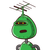# if the roots of the equation 6 x square – 13 x + m equal to zero receive true of each other find the value of m​

if the roots of the equation 6 x square – 13 x + m equal to zero receive true of each other find the value of m​

### 1 thought on “if the roots of the equation 6 x square – 13 x + m equal to zero receive true of each other find the value of m​”

1.Quadratic Equation  6x² – 13x + m= 0

Roots are reciprocal to each other

To Find : Value of m

m ની કિંમત શોધો.​

Solution:

6x² – 13x + m= 0

Roots are reciprocal to each other

Let say α , 1/α

=> Product of Roots =  α * 1/α  =  1

Product of Roots = m/6

m/6 = 1

=> m = 6

Value of m = 6